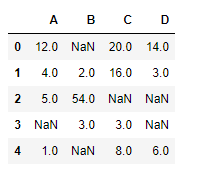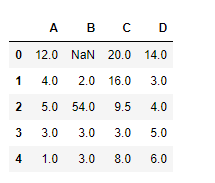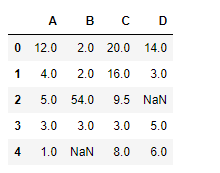# Pandas dataframe.interpolate()

## Python | Pandas dataframe.interpolate()

Python is a great language for doing data analysis, primarily because of the fantastic ecosystem of data-centric python packages. Pandas is one of those packages and makes importing and analyzing data much easier.

Pandas` dataframe.interpolate()` function is basically used to fill `NA` values in the dataframe or series. But, this is a very powerful function to fill the missing values. It uses various interpolation technique to fill the missing values rather than hard-coding the value.

Syntax: DataFrame.interpolate(method=’linear’, axis=0, limit=None, inplace=False, limit_direction=’forward’, limit_area=None, downcast=None, **kwargs)

Parameters :
method : {‘linear’, ‘time’, ‘index’, ‘values’, ‘nearest’, ‘zero’, ‘slinear’, ‘quadratic’, ‘cubic’, ‘barycentric’, ‘krogh’, ‘polynomial’, ‘spline’, ‘piecewise_polynomial’, ‘from_derivatives’, ‘pchip’, ‘akima’}

axis : 0 fill column-by-column and 1 fill row-by-row.
limit : Maximum number of consecutive NaNs to fill. Must be greater than 0.
limit_direction : {‘forward’, ‘backward’, ‘both’}, default ‘forward’
limit_area : None (default) no fill restriction. `inside `Only fill NaNs surrounded by valid values (interpolate). `outside `Only fill NaNs outside valid values (extrapolate). If limit is specified, consecutive NaNs will be filled in this direction.
inplace : Update the NDFrame in place if possible.
downcast : Downcast dtypes if possible.
kwargs : keyword arguments to pass on to the interpolating function.

Returns : Series or DataFrame of same shape interpolated at the NaNs

Example #1: Use `interpolate()` function to fill the missing values using linear method.

 `# importing pandas as pd` `import` `pandas as pd` ` ` `# Creating the dataframe ` `df ``=` `pd.DataFrame({``"A"``:[``12``, ``4``, ``5``, ``None``, ``1``],` `                   ``"B"``:[``None``, ``2``, ``54``, ``3``, ``None``],` `                   ``"C"``:[``20``, ``16``, ``None``, ``3``, ``8``],` `                   ``"D"``:[``14``, ``3``, ``None``, ``None``, ``6``]})` ` ` `# Print the dataframe` `df`Let’s interpolate the missing values using Linear method. Note that Linear method ignore the index and treat the values as equally spaced.

 `# to interpolate the missing values` `df.interpolate(method ``=``'linear'``, limit_direction ``=``'forward'``)`

Output :As we can see the output, values in the first row could not get filled as the direction of filling of values is `forward` and there is no previous value which could have been used in interpolation.
Example #2: Use `interpolate()` function to interpolate the missing values in the backward direction using linear method and putting a limit on maximum number of consecutive `Na` values that could be filled.

 `# importing pandas as pd` `import` `pandas as pd` ` ` `# Creating the dataframe ` `df ``=` `pd.DataFrame({``"A"``:[``12``, ``4``, ``5``, ``None``, ``1``],` `                   ``"B"``:[``None``, ``2``, ``54``, ``3``, ``None``],` `                   ``"C"``:[``20``, ``16``, ``None``, ``3``, ``8``],` `                   ``"D"``:[``14``, ``3``, ``None``, ``None``, ``6``]})` ` ` `# to interpolate the missing values` `df.interpolate(method ``=``'linear'``, limit_direction ``=``'backward'``, limit ``=` `1``)`

Output :Notice the fourth column, only one missing value has been filled as we have put the limit to 1. The missing value in the last row could not get filled as no row exists after that from which the value could be interpolated.

Last Updated on March 17, 2022 by admin

## Opencv Python program for Face DetectionOpencv Python program for Face Detection

Opencv Python program for Face Detection The objective of the program given is to detect

## Python String isspace() MethodPython String isspace() Method

Python String isspace() Method Python String isspace() is a built-in method used for string handling. The isspace()

## Comprehensions in PythonComprehensions in Python

Comprehensions in Python Comprehensions in Python provide us with a short and concise way to

Difference between input() and sys.stdin.readline() Python is a widely used general-purpose language that can be

## Python program to find the sum of all items in a dictionaryPython program to find the sum of all items in a dictionary

Python program to find the sum of all items in a dictionary Given a dictionary

## numpy.sqrt() in Pythonnumpy.sqrt() in Python

numpy.sqrt() in Python numpy.sqrt(array[, out]) function is used to determine the positive square-root of an array,

## Python | Linear Regression using sklearnPython | Linear Regression using sklearn

Python | Linear Regression using sklearn Linear Regression is a machine learning algorithm based on

## Django ORM – Inserting, Updating & Deleting DataDjango ORM – Inserting, Updating & Deleting Data

Django ORM – Inserting, Updating & Deleting Data Django lets us interact with its database